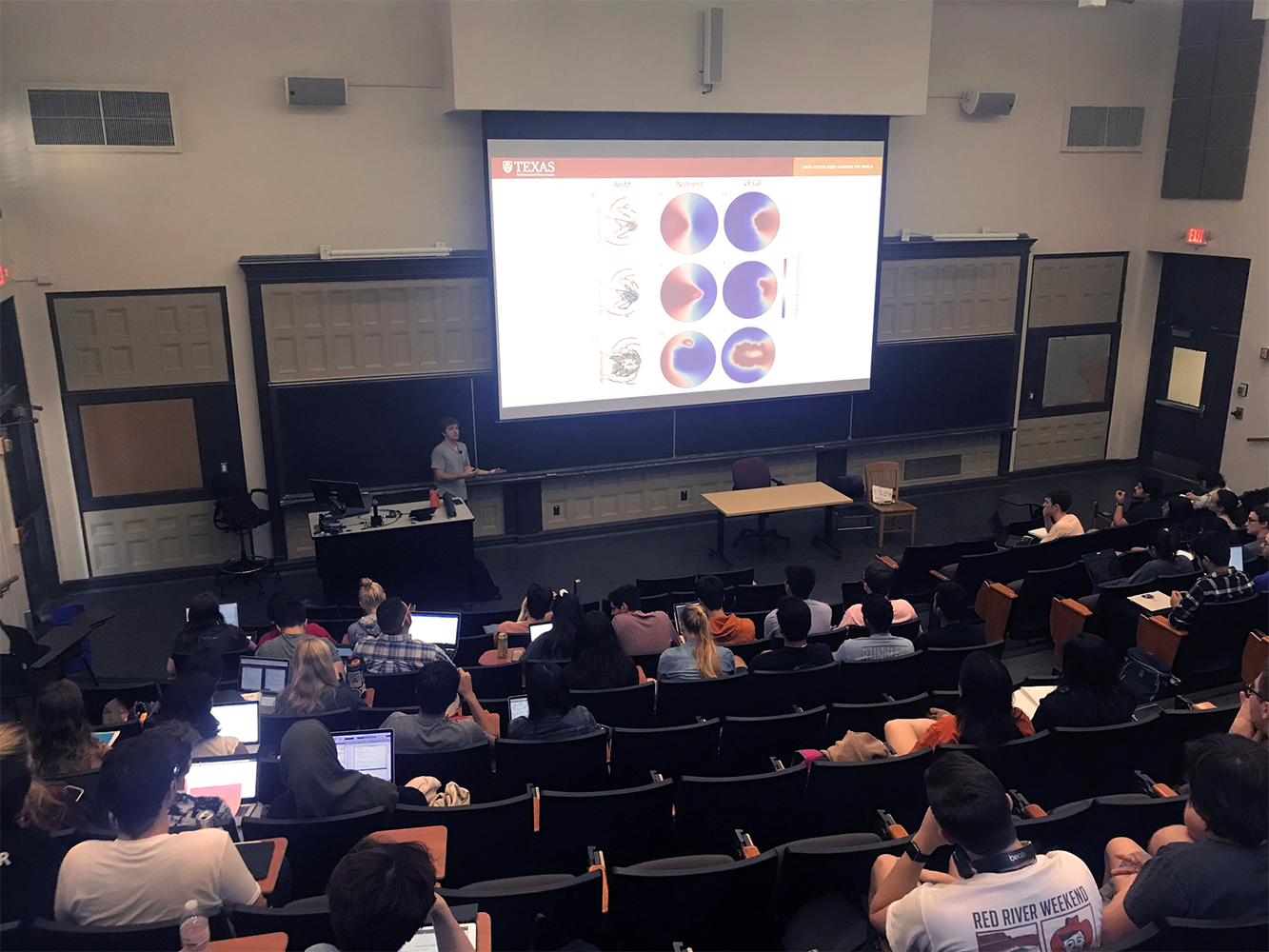CCO member Caleb Philips recently gave two guest lectures to undergraduate BME students in introduction to computing and numerical methods.

Caleb focused on how the numerical and computation techniques current undergraduates are learning can be applied to study biological phenomena through mathematical models. Caleb also introduced the students to how these mathematical modes to make predictions, model calibration, and model validation. Caleb concluded with introducing the students to the types of biological measurements he uses to calibrate his own models.